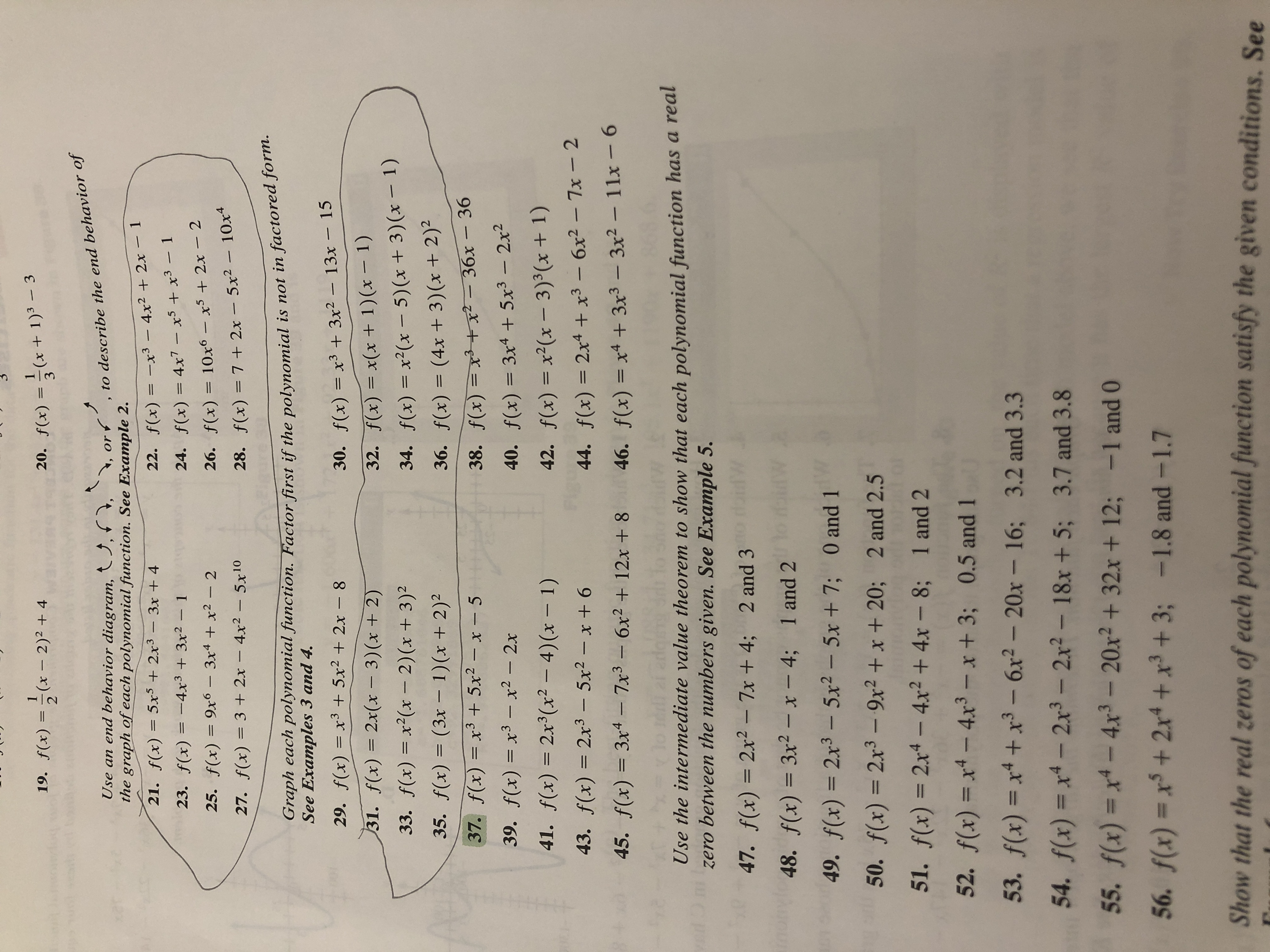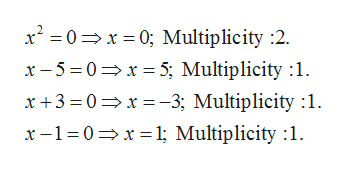# 19. f(x)(x- 2)2 + 4x + 1)3 - 3320. f(x)Use an end behavior diagram, , , , orthe graph of each polynomial function. See Example 2.to describe the end behavior off(x)= 5x5 +2x3-3x + 421.22. f(x)-x3 - 4x2 + 2x- 1123. f(x) = -4x3 + 3x2- 124. f(x) = 4x7 - x5 x325. f(x) = 9x6- 3x4 +x2 - 226. f(x) 10x6 - x5 + 2x - 227. f(x) = 3 + 2x- 4x2- 5x10f(x) = 7 2x- 5x2- 10x4Graph each polynomial function. Factor first if the polynomial is not in factored form.See Examples 3 and 4.29. f(x)= xr3 +5x2 +2x - 830. f(x)= x3 +3x2- 13x - 1531. f(x) 2x(x - 3) (x + 2)33. f(x) x2(x- 2) (x + 3)232. f(x) x(x + 1)(x - 1)34. f(x) x2(x- 5) (x + 3) (x - 1)36. f(x) (4x + 3) (x + 2)235. f(x) (3x- 1)(x + 2)237. f(x) x3+ 5x2 - x - 538. f(x) x3-+x- 36x- 3639. f(x) x3 - x2 - 2x140. f(x) 3x4 +5x3-2x241. f(x) 2x3(x? - 4) (x - 1)42. f(x) x2(x- 3)'(x + 1)43. f(x)2x3 - 5x2 - x + 644. f(x) 2x4 + x3 -6x2- 7x - 2145. f(x) = 3x4 7x3-6x2 +12x + 846. f(x) = x4 +3x3- 3x2- 11x - 6Use the intermediate value theorem to show that each polynomial function has a recalbetween the numbers given. See Example 5.yer47. f(x) 2x2-7x+ 4; 2 and 348. f(x) 3r2-x- 4; l and49. f (x) = 2x3 - 5x2 -5x+7; 0 and 150. f(x) 2x3-9x2+x+ 20; 2 and 2.51151. f(x) 2x4- 4x2 + 4x- 8;1 and 252. f(x) x4-4x3 - x + 3; 0.5 and 153. f(x) x4+x3 -6x2-20x - 16; 3.2 and 3.354. f(x) x4-2x3-2x2- 18x +5; 3.7 and 3.855. f(x) = x4-4r3-20x2+32x + 12; -1 and 056. f(x) = x5 + 2xrt +x3+3;-1.8 and-1.7Show that the real zeros of each polynomial function satisfy the given conditions. See

Question
8 views

34.help_outlineImage Transcriptionclose19. f(x)(x- 2)2 + 4 x + 1)3 - 3 3 20. f(x) Use an end behavior diagram, , , , or the graph of each polynomial function. See Example 2. to describe the end behavior of f(x)= 5x5 +2x3-3x + 4 21. 22. f(x) -x3 - 4x2 + 2x- 1 1 23. f(x) = -4x3 + 3x2- 1 24. f(x) = 4x7 - x5 x3 25. f(x) = 9x6- 3x4 +x2 - 2 26. f(x) 10x6 - x5 + 2x - 2 27. f(x) = 3 + 2x- 4x2 - 5x10 f(x) = 7 2x- 5x2- 10x4 Graph each polynomial function. Factor first if the polynomial is not in factored form. See Examples 3 and 4. 29. f(x)= xr3 +5x2 +2x - 8 30. f(x)= x3 +3x2- 13x - 15 31. f(x) 2x(x - 3) (x + 2) 33. f(x) x2(x- 2) (x + 3)2 32. f(x) x(x + 1)(x - 1) 34. f(x) x2(x- 5) (x + 3) (x - 1) 36. f(x) (4x + 3) (x + 2)2 35. f(x) (3x- 1)(x + 2)2 37. f(x) x3+ 5x2 - x - 5 38. f(x) x3-+x- 36x- 36 39. f(x) x3 - x2 - 2x 1 40. f(x) 3x4 +5x3-2x2 41. f(x) 2x3(x? - 4) (x - 1) 42. f(x) x2(x- 3)'(x + 1) 43. f(x) 2x3 - 5x2 - x + 6 44. f(x) 2x4 + x3 -6x2- 7x - 2 1 45. f(x) = 3x4 7x3-6x2 +12x + 8 46. f(x) = x4 +3x3- 3x2- 11x - 6 Use the intermediate value theorem to show that each polynomial function has a recal between the numbers given. See Example 5. yer 47. f(x) 2x2-7x+ 4; 2 and 3 48. f(x) 3r2-x- 4; l and 49. f (x) = 2x3 - 5x2 -5x+7; 0 and 1 50. f(x) 2x3-9x2+x+ 20; 2 and 2.5 11 51. f(x) 2x4- 4x2 + 4x- 8; 1 and 2 52. f(x) x4-4x3 - x + 3; 0.5 and 1 53. f(x) x4+x3 -6x2-20x - 16; 3.2 and 3.3 54. f(x) x4-2x3-2x2- 18x +5; 3.7 and 3.8 55. f(x) = x4-4r3-20x2+32x + 12; -1 and 0 56. f(x) = x5 + 2xrt +x3+3; -1.8 and-1.7 Show that the real zeros of each polynomial function satisfy the given conditions. See fullscreen
check_circle

Step 1

Degree of the given ploynomial is : 5, which is odd and

Leading coefficent is : 1, which is a positive number.

Step 2

According to rules of end behaviour of a Polynomila function, the polynomila with odd degree and positive leading coefficent would " go down on the left and go up on the right".

Step 3

Now, let us find the zeros of the given polynomila with their multi...help_outlineImage Transcriptionclosex20x 0; Multiplicity :2 x-50x 5; Multiplicity :1 x+3 0 x-3; Multiplicity :1 x-1-0 xl; Multiplicity 1 fullscreen

### Want to see the full answer?

See Solution

#### Want to see this answer and more?

Solutions are written by subject experts who are available 24/7. Questions are typically answered within 1 hour.*

See Solution
*Response times may vary by subject and question.
Tagged in

### Polynomials